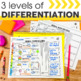# 1st Grade Math Spiral Review | 1st Grade Homework | Distance LearningSubject
Resource Type
File Type

Zip

(64 MB|242 pages)
Standards
4 Products in this Bundle
4 products
1. This 1st grade resource is perfect for morning work, homework, daily math review, and independent math centers. 1st grade spiral math review for first quarter includes 10 weeks of spiral math sheets with 3 differentiated levels and answer keys. What's Included in 1st Quarter Spiral Math Review She
2. This 1st grade resource is perfect for morning work, homework, daily math review, and independent math centers. 1st grade spiral math review for second quarter includes 10 weeks of spiral math sheets with 3 differentiated levels and answer keys. What's Included in 2nd Quarter Spiral Math Review
3. This 1st grade resource is perfect for morning work, homework, daily math review, and independent math centers.1st grade spiral math review for third quarter includes 10 weeks of spiral math sheets with 3 differentiated levels and answer keys. What's Included in 3rd Quarter Spiral Math Review Sheet
4. This 1st grade resource is perfect for morning work, homework, daily math review, and independent math centers. 1st grade spiral math review for fourth quarter includes 10 weeks of spiral math sheets with 3 differentiated levels and answer keys. What's Included in 4th Quarter Spiral Math Review
• Bundle Description
• StandardsNEW

This 1st grade resource WITH 3 LEVELS OF DIFFERENTIATION is perfect for morning work, homework, daily math review, and independent math centers.

1st grade spiral math review for the ENTIRE YEAR includes 40 weeks of spiral math sheets with answer keys.

What's Included in the Spiral Math Review Sheets BUNDLE:

►At A Glance Sheet

►40 Weeks of Spiral Math Review Sheets

►3 Levels of Differentiation: BELOW, AT, and ABOVE GRADE LEVEL

►Common Core aligned

►Detailed explanation of how to use the resource

Skills included in the Spiral Math Review Sheets BUNDLE:

FIRST QUARTER:

►Kindergarten Review (4 weeks)

►Counting to 120 (2 weeks)

►Shape Attributes

►Compose 2D and 3D Shapes

►Partition Circles and Rectangles

►Mixed Review of 1st Quarter Concepts

SECOND QUARTER:

►Relate Counting to Subtraction

►Subtract Within 20

►Mixed Addition & Subtraction Within 20

►Order Objects by Length

►Express the Length of an Object

►Time to the Hour

►Time to the Half Hour

►Mixed Review of 2nd Quarter Concepts

THIRD QUARTER:

►Organize & Represent Data

►Find Unknown Number in an Equation

►Subtract Within 20 Word Problems

►Understand the Meaning of an Equal Sign

►Addition Word Problems with 3 Numbers

►Interpret Data

►Mixed Addition & Subtraction Word Problems

►Mixed Review of 3rd Quarter Concepts

FOURTH QUARTER:

►Place Value (2 weeks)

►Compare 2-Digit Numbers

►Mental Math: 10 More and 10 Less

►Subtract Multiples of 10

►Mixed Review of ALL 1st Grade Concepts (4 weeks)

▼▼▼▼▼▼▼▼▼▼▼▼▼▼▼▼▼▼▼▼▼▼▼▼▼▼▼▼▼▼▼▼▼▼▼▼▼▼▼▼▼▼▼▼

If you would like to get updates on NEW and CURRENT resources...

▼▼▼▼▼▼▼▼▼▼▼▼▼▼▼▼▼▼▼▼▼▼▼▼▼▼▼▼▼▼▼▼▼▼▼▼▼▼▼▼▼▼▼▼

Permission to copy for single classroom use only.

Electronic distribution limited to single classroom use only.

Not for public display.

Partition circles and rectangles into two and four equal shares, describe the shares using the words halves, fourths, and quarters, and use the phrases half of, fourth of, and quarter of. Describe the whole as two of, or four of the shares. Understand for these examples that decomposing into more equal shares creates smaller shares.
Compose two-dimensional shapes (rectangles, squares, trapezoids, triangles, half-circles, and quarter-circles) or three-dimensional shapes (cubes, right rectangular prisms, right circular cones, and right circular cylinders) to create a composite shape, and compose new shapes from the composite shape.
Distinguish between defining attributes (e.g., triangles are closed and three-sided) versus non-defining attributes (e.g., color, orientation, overall size); build and draw shapes to possess defining attributes.
Determine the unknown whole number in an addition or subtraction equation relating three whole numbers. For example, determine the unknown number that makes the equation true in each of the equations 8 + ? = 11, 5 = ▯ - 3, 6 + 6 = ▯.
Understand the meaning of the equal sign, and determine if equations involving addition and subtraction are true or false. For example, which of the following equations are true and which are false? 6 = 6, 7 = 8 - 1, 5 + 2 = 2 + 5, 4 + 1 = 5 + 2.
Total Pages
242 pages
Included
Teaching Duration
1 Year
Report this Resource to TpT
Reported resources will be reviewed by our team. Report this resource to let us know if this resource violates TpT’s content guidelines.